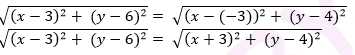Newbie

# Find a relation between x and y such that the point (x, y) is equidistant from the point (3, 6) and (- 3, 4). Q.10

• 0

Give me the best solution of coordinate geometry of  ncert 10 class . How i solve this question fast and easy way  please help me to solve this important question. Find a relation between x and y such that the point (x, y) is equidistant from the point (3, 6) and (- 3, 4).

Share

1. Point (x, y) is equidistant from (3, 6) and ( – 3, 4).Squaring both sides, (x – 3)2+(y – 6)2 = (x + 3)2 +(y – 4)2

x+ 9 – 6x + y2+ 36 – 12y = x+ 9 + 6x + y+16 – 8y

36 – 16 = 6x + 6x + 12y – 8y

20 = 12x + 4y

3x + y = 5

3x + y – 5 = 0

• 0